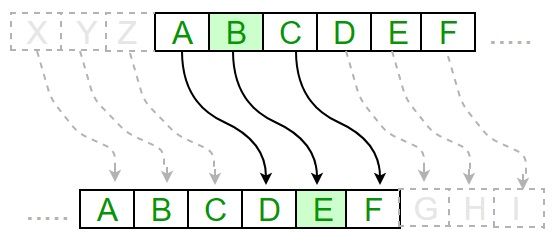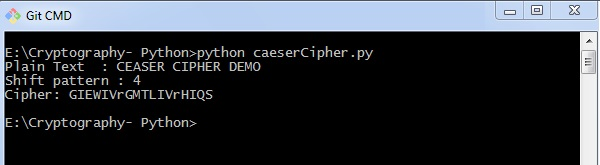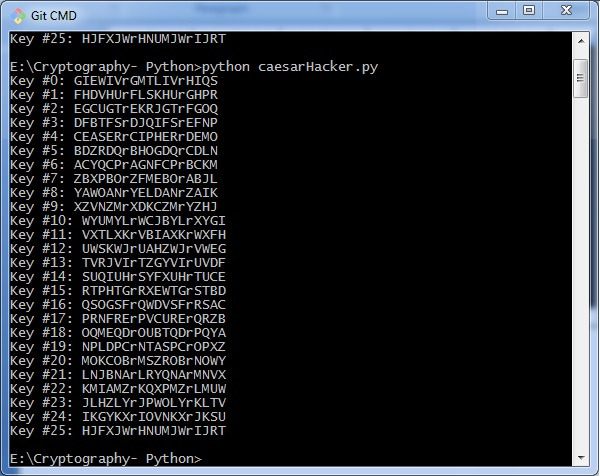# Cryptography with Python – Caesar Cipher

In the last chapter, we have dealt with reverse cipher. This chapter talks about Caesar cipher in detail.

## Algorithm of Caesar Cipher

The algorithm of Caesar cipher holds the following features −

• Caesar Cipher Technique is the simple and easy method of encryption technique.

• It is simple type of substitution cipher.

• Each letter of plain text is replaced by a letter with some fixed number of positions down with alphabet.

The following diagram depicts the working of Caesar cipher algorithm implementation −The program implementation of Caesar cipher algorithm is as follows −

```def encrypt(text,s):
result = ""
# transverse the plain text
for i in range(len(text)):
char = text[i]
# Encrypt uppercase characters in plain text

if (char.isupper()):
result += chr((ord(char) + s-65) % 26 + 65)
# Encrypt lowercase characters in plain text
else:
result += chr((ord(char) + s - 97) % 26 + 97)
return result
#check the above function
text = "CEASER CIPHER DEMO"
s = 4

print "Plain Text : " + text
print "Shift pattern : " + str(s)
print "Cipher: " + encrypt(text,s)```

### Output

You can see the Caesar cipher, that is the output as shown in the following image −### Explanation

The plain text character is traversed one at a time.

• For each character in the given plain text, transform the given character as per the rule depending on the procedure of encryption and decryption of text.

• After the steps is followed, a new string is generated which is referred as cipher text.

## Hacking of Caesar Cipher Algorithm

The cipher text can be hacked with various possibilities. One of such possibility is Brute Force Technique, which involves trying every possible decryption key. This technique does not demand much effort and is relatively simple for a hacker.

The program implementation for hacking Caesar cipher algorithm is as follows −

```message = 'GIEWIVrGMTLIVrHIQS' #encrypted message
LETTERS = 'ABCDEFGHIJKLMNOPQRSTUVWXYZ'

for key in range(len(LETTERS)):
translated = ''
for symbol in message:
if symbol in LETTERS:
num = LETTERS.find(symbol)
num = num - key
if num < 0:
num = num + len(LETTERS)
translated = translated + LETTERS[num]
else:
translated = translated + symbol
print('Hacking key #%s: %s' % (key, translated))```

Consider the cipher text encrypted in the previous example. Then, the output with possible hacking methods with the key and using brute force attack technique is as follows −